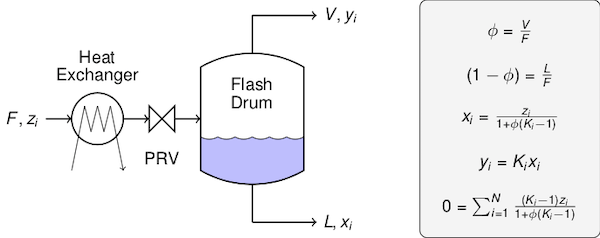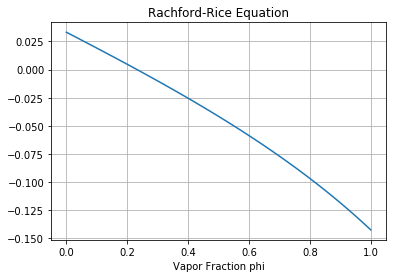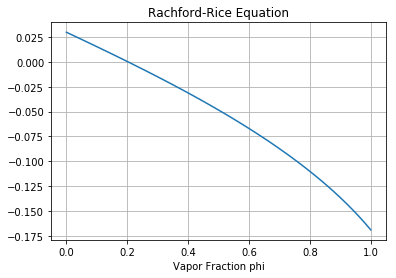This notebook contains course material from CBE20255 by Jeffrey Kantor (jeff at nd.edu); the content is available on Github. The text is released under the CC-BY-NC-ND-4.0 license, and code is released under the MIT license.

# Isothermal Flash and the Rachford-Rice Equation¶

## Summary¶

This Jupyter notebook illustrates the use of the Rachford-Rice equation solve the material balances for an isothermal flash of an ideal mixture. The video is used with permission from learnCheme.com, a project at the University of Colorado funded by the National Science Foundation and the Shell Corporation.

## Derivation of the Rachford-Rice Equation¶

The derivation of the Rachford-Rice equation is a relatively straightford application of component material balances and Raoult's law for an ideal solution.

In :
from IPython.display import YouTubeVideo

Out:

The quantities, definitions, and equations are summarized in the following figure.To sketch the derivation, we begin with the overall constraint on the liquid phase and vapor phase mole fractions $x_1 + x_2 + \cdots + x_N = 1$ and $y_1 + y_2 + \cdots + y_N = 1$. Subtracting the first from the second we find

$$\sum_{n=1}^N (y_n - x_n) = 0$$

This doesn't look like much, but it turns out to be the essential trick in the development.

Next we need expressions for $y_n$ and $x_n$ to substitute into terms in the sum. We get these by solving the component material balance and equilibrium equations for $y_n$ and $x_n$. For each species we write a material balance

$$L x_n + V y_n = F z_n$$

Dividing through by the feedrate we get a parameter $\phi = \frac{V}{L}$ denoting the fraction of the feedstream that leaves the flash unit in the vapor stream, the remaining fraction $1-\phi$ leaving in the liquid stream. With this notation the material balance becomes

$$(1-\phi)x_n + \phi y_n = z_n$$

for each species.

The second equation is

$$y_n = K_n x_n$$

where the 'K-factor' for an ideal solution is given by Raoult's law

$$K_n = \frac{P_n^{sat}(T)}{P}$$

The K-factor depends on the operating pressure and temperature of the flash unit. Solving the material balance and equilibrium equations gives

$$x_n = \frac{z_n}{1 + \phi(K_n - 1)}$$$$y_n = \frac{K_n z_n}{1 + \phi(K_n - 1)}$$

so that the difference $y_n - x_n$ is given by

$$y_n - x_n = \frac{(K_n - 1)z_n}{1 + \phi(K_n - 1)}$$

Substitution leads to the Rachford-Rice equation

$$\sum_{n=1}^{N} \frac{(K_n - 1)z_n}{1 + \phi(K_n - 1)} = 0$$

This equation can be used to solve a variety of vapor-liquid equilibrium problems as outline in the following table.

## Problem Classification¶

Problem Type zi's T P φ Action
Bubble Point unknown 0 Set xi = zi. Solve for T and yi's
Bubble Point unknown 0 Set xi = zi. Solve for P and yi's
Dew Point unknown 1 Set yi = zi. Solve for T and xi's
Dew Point unknown 1 Set yi = zi. Solve for P and xi's
Isothermal Flash unknown Solve for φ, xi's, and yi's

## Isothermal Flash of a Binary Mixture¶

Problem specifications

In :
import numpy as np
import matplotlib.pyplot as plt
%matplotlib inline

In :
A = 'acetone'
B = 'ethanol'

P = 760
T = 65

z = dict()
z[A] = 0.6
z[B] = 1 - z[A]


Compute the K-factors for the given operating conditions

In :
# Antoine's equations. T [deg C], P [mmHg]
Psat = dict()
Psat[A] = lambda T: 10**(7.02447 - 1161.0/(224 + T))
Psat[B] = lambda T: 10**(8.04494 - 1554.3/(222.65 + T))

# Compute K-factors
K = dict()
K[A] = Psat[A](T)/P
K[B] = Psat[B](T)/P

print("Pressure     {:6.2f} [mmHg]".format(P))
print("Temperature  {:6.2f} [deg C]".format(T))
print("K-factors:")
for n in Psat:
print("   {:s}  {:7.3f}".format(n,K[n]))

Pressure     760.00 [mmHg]
Temperature   65.00 [deg C]
K-factors:
acetone    1.338
ethanol    0.576


Rachford-Rice equation

In :
def RR(phi):
return (K[A]-1)*z[A]/(1 + phi*(K[A]-1)) + (K[B]-1)*z[B]/(1 + phi*(K[B]-1))

phi = np.linspace(0,1)
plt.plot(phi,[RR(phi) for phi in phi])
plt.xlabel('Vapor Fraction phi')
plt.title('Rachford-Rice Equation')
plt.grid();Finding roots of the Rachford-Rice equation

In :
from scipy.optimize import brentq

phi = brentq(RR,0,1)

print("Vapor Fraction  {:6.4f}".format(phi))
print("Liquid Fraction {:6.4f}".format(1-phi))

Vapor Fraction  0.2317
Liquid Fraction 0.7683


Compositions

In :
x = dict()
y = dict()

print("Component    z[n]    x[n]    y[n]")

for n in [A,B]:
x[n] = z[n]/(1 + phi*(K[n]-1))
y[n] = K[n]*x[n]
print("{:10s} {:6.4n}  {:6.4f}  {:6.4f}".format(n,z[n],x[n],y[n]))

Component    z[n]    x[n]    y[n]
acetone       0.6  0.5565  0.7444
ethanol       0.4  0.4435  0.2556


## Multicomponent Mixtures¶

In :
P = 760
T = 65

z = dict()
z['acetone'] = 0.6
z['benzene'] = 0.01
z['toluene'] = 0.01
z['ethanol'] = 1 - sum(z.values())

In :
Psat = dict()
Psat['acetone'] = lambda T: 10**(7.02447 - 1161.0/(224 + T))
Psat['benzene'] = lambda T: 10**(6.89272 - 1203.531/(219.888 + T))
Psat['ethanol'] = lambda T: 10**(8.04494 - 1554.3/(222.65 + T))
Psat['toluene'] = lambda T: 10**(6.95805 - 1346.773/(219.693 + T))

K = {n : lambda P,T,n=n: Psat[n](T)/P for n in Psat}

print("Pressure     {:6.2f} [mmHg]".format(P))
print("Temperature  {:6.2f} [deg C]".format(T))
print("K-factors:")
for n in K:
print("   {:s}  {:7.3f}".format(n,K[n](P,T)))

Pressure     760.00 [mmHg]
Temperature   65.00 [deg C]
K-factors:
acetone    1.338
benzene    0.613
ethanol    0.576
toluene    0.222

In :
def RR(phi):
return sum([(K[n](P,T)-1)*z[n]/(1 + phi*(K[n](P,T)-1)) for n in K.keys()])

phi = np.linspace(0,1)
plt.plot(phi,[RR(phi) for phi in phi])
plt.xlabel('Vapor Fraction phi')
plt.title('Rachford-Rice Equation')
plt.grid();In :
from scipy.optimize import brentq

phi = brentq(RR,0,1)

print("Vapor Fraction  {:6.4f}".format(phi))
print("Liquid Fraction {:6.4f}".format(1-phi))

Vapor Fraction  0.2033
Liquid Fraction 0.7967

In :
x = {n: z[n]/(1 + phi*(K[n](P,T)-1)) for n in z}
y = {n: K[n](P,T)*z[n]/(1 + phi*(K[n](P,T)-1)) for n in z}

print("Component    z[n]    x[n]    y[n]")

for n in z.keys():
print("{:10s} {:6.4f}  {:6.4f}  {:6.4f}".format(n,z[n],x[n],y[n]))

Component    z[n]    x[n]    y[n]
acetone    0.6000  0.5615  0.7511
benzene    0.0100  0.0109  0.0067
toluene    0.0100  0.0119  0.0026
ethanol    0.3800  0.4158  0.2396


Experiments suggest the bursting pressure of a 2 liter soda bottle is 150 psig.

## Exercises¶

### Design of a Carbonated Beverage¶

The purpose of carbonating beverages is to provide a positive pressure inside the package to keep out oxygen and other potential contaminants. The burst pressure of 2 liter soda bottles has been measured to be 150 psig (approx. 10 atm). For safety, suppose you want the bottle pressure to be no more than 6 atm gauge on a hot summer day in Arizona (say 50 °C, ) and yet have at least 0.5 atm of positive gauge pressure at 0 °C. Assuming your beverage is a mixture of CO2 and water, is it possible to meet this specification? What concentration (measured in g of CO2 per g of water) would you recommend?

In [ ]: